Articles published in Geometry

Trick-or-Treaters Are Using the Executive MindsetHere’s Why You’re Getting Geometry Problems on GMAT Data Sufficiency WrongDid you know that you can attend the first session of any of our online or in-person GMAT courses absolutely free? We’re not kidding! Check out our upcoming courses here.

I had a student recently whose Data Sufficiency (DS) accuracy was generally very high, and whose knowledge of geometry rules was solid. And yet, she was getting almost every geometry DS question wrong on practice tests!

This is actually very common: students who are otherwise good at geometry and/or DS struggle when the two things are put together.

Here are the 3 main reasons that students miss geometry DS problems: Read more

Manhattan Prep’s GMAT® study app is now available!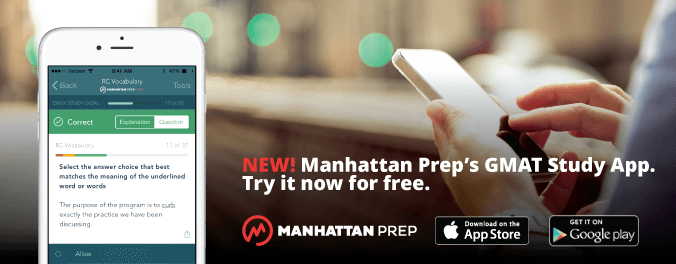I am very excited to announce that our new GMAT® study app is available on both iOS and Android!

Geometry – Who Said Geometry? It’s Algebra!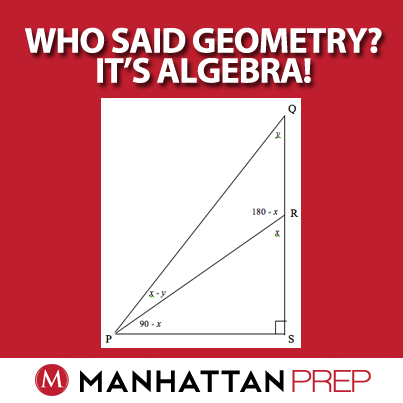The GMAT quant section has many faces – there are a number of content areas, and it is best to try to master as many of them as you can before test day. It is important, however, that you not compartmentalize too much. In many of the harder questions in fact, two or more topics often show up together. You can easily find quadratics in a consecutive integer question, coordinate geometry in a probability question, number properties in a function question, for example. One common intersection of two topics that I find surprises many students is that of geometry and algebra. Many people expect a geometry question to be about marking up diagrams with values or tick marks to show equality and/or applying properties and formulas to calculate or solve. While these are no doubt important skill sets in geometry, don’t forget to pull out one of the most important skills from your  GMAT tool bag – the almighty variable! x’s and y’s have a welcomed home in many a geometry question, though you might find that you are the one who has to take the initiative to put them there!

Take a look at this data sufficiency question from GMATPrep®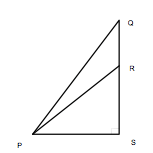In the figure shown, the measure ofPRS is how many degrees greater than the measure ofPQR?

(1) The measure ofQPR is 30 degrees.

(2) The sum of the measures ofPQR andPRQ is 150 degrees.

How did you do? Don’t feel bad if you’re a little lost on this one. This is a difficult question, though you’ll see that with the right moves it is quite doable. At the end of this discussion, you’ll even see how you could put up a good guess on this one.

As is so often the case in a data sufficiency question, the right moves here start with the stem – in rephrasing the question. Unfortunately the stem doesn’t appear to provide us with a lot of given information. As indicated in the picture, you have a 90 degree angle atPQR and that seems like all that you are given, but it’s not! There are some other inherent RELATIONSHIPS, ones that are implied by the picture. For examplePRS andPRQ sum to 180 degrees. The problem, however, is how do you CAPTURE THOSE RELATIONSHIPS? The answer is simple – you capture those relationships the way you always capture relationships in math when the relationship is between two unknown quantities – you use variables!

But where should you put the variables and how many variables should you use?  This last question is one that you’ll likely find yourself pondering  a number of times on the GMAT. Some believe the answer to be a matter of taste. My thoughts are always use as few variables as possible. If you can capture all of the relationships that you want to capture with one variable, great. If you need two variables, so be it. The use of three or more variables would be rather uncommon in a geometry question, though you could easily see that in a word problem.  Keep one thing in mind when assigning variables: the more variables you use, usually the more equations you will need to write in order to solve.

As for the first question above about where to place the variables, you can take a closer look in this question at what they are asking and use that as a guide. They ask for the   (degree) difference betweenPRS andPQR. SincePRS is in the question, start by labelingPRS as x. SincePRS andPRQ sum to 180 degrees, you can also labelPRQ as (180 – x) andRPS as (180 – x – 90) or (90 – x).

Can you continue to label the other angles in triangle PRQ in terms of x or is it now time to place a second variable, y? Since you still have two other unknown quantities in that triangle, it’s in fact time for that y. The logical place of where to put it is onPQR since that is also part of the actual question. The temptation is to stop there – DON’T! Continue to label the final angle of the triangle,QPR, using your newfound companions, x and y.QPR can be labeled as [180 – y – (180 – x)] or (xy). Now all of the angles in the triangle are labeled and you are poised and ready to craft an algebraic equation/expression to capture any other relationships that might come your way.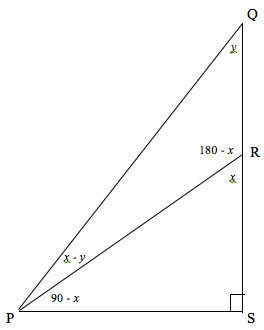Before you rush off to the statements, however, there is one last step. Formulate what the question is really asking in terms of x and y. The question rephrases to “What is the value of xy?”

Now you can finally head to the statements. Oh the joy of a fully dissected data sufficiency stem – 90% of the work has already been done!

Statement (1) tells you that the measure ofQPR is 30 degrees. Using your xy expression from the newly labeled diagram as the value ofQPR, you can jot down the equation xy = 30. Mission accomplished! The statement is sufficient to answer the question “what is the value of xy?”

Statement (2) indirectly provides the same information as statement (1). If the two other angles of triangle PQR sum to 150 degrees, thenQPR is 30 degrees, so the statement is sufficient as well. If you somehow missed this inference and instead directly pulled from the diagram y + (180 – x) and set that equal to 150, you’d come to the same conclusion. Either way the algebra saves the day!

The answer to the question is D, EACH statement ALONE is sufficient to answer the question asked.

NOTE here that from a strategic guessing point of view, noticing that statements (1) and (2) essentially provide the same information allows you to eliminate answer choices A, B and C: A and B because how could it be one and not the other if they are the same, and C because there is nothing gained by combining them if they provide exactly the same information.

The takeaways from this question are as follows:

(1) When a geometry question has you staring at the diagram, uncertain of how to proceed in marking things up or capturing relationships that you know exist – use variables! Those variables will help you move through the relationships just as actual values would.

(2) In data sufficiency geometry questions, when possible represent the question in algebraic form so the target becomes clear and so that the rules of algebra are there to help you assess sufficiency.

(3) Once you have assigned a variable, continue to label as much of the diagram in terms of that variable. If you need a second variable to fully label the diagram, use it. If you can get away with just one variable and still accomplish the mission, do so.

Most GMAT test-takers know that they need to develop clear strategies when it comes to different types of word problems, and most of those involve either muscling your way through the problem with some kind of practical approach (picking numbers, visualizing, back-solving, logical reasoning) or writing out algebraic equations and solving.  There are of course pluses and minuses to all of the approaches and those need to be weighed by each person on an individual basis. What few realize, however, is that geometry questions can also demonstrate that level of complexity and thus can often also be solved with the tools of algebra. When actual values are few and far between, don’t hesitate to pull out an “x” (and possibly also a “y”) and see what kind of equations/expressions you can cook up.

For more practice in “algebrating” a geometry question, please see OG 13th DS 79 and Quant Supplement 2nd editions PS 157, 162 and DS 60, 114 and 123.

3 Steps to Better GeometryA couple of months ago, we talked about what to do when a geometry problem pops up on the screen. Do you remember the basic steps? Try to implement them on the below GMATPrep® problem from the free tests.

* ”In the xy-plane, what is the y-intercept of line L?

“(1) The slope of line L is 3 times its y-intercept
“(2) The x-intercept of line L is – 1/3”

My title (3 Steps to Better Geometry) is doing double-duty. First, here’s the general 3-step process for any quant problem, geometry included: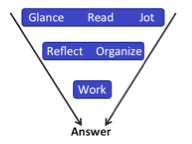All geometry problems also have three standard strategies that fit into that process.

First, pick up your pen and start drawing! If they give you a diagram, redraw it on your scrap paper. If they don’t (as in the above problem), draw yourself a diagram anyway. This is part of your Glance-Read-Jot step.

Second, identify the “wanted” element and mark this element on your diagram. You’ll do this as part of the Glance-Read-Jot step, but do it last so that it leads you into the Reflect-Organize stage. Where am I trying to go? How can I get there?

Third, start Working! Infer from the given information. Geometry on the GMAT can be a bit like the proofs that we learned to do in high school. You’re given a couple of pieces of info to start and you have to figure out the 4 or 5 steps that will get you over to the answer, or what you’re trying to “prove.”

Let’s dive into this problem. They’re talking about a coordinate plane, so you know the first step: draw a coordinate plane on your scrap paper. The question indicates that there’s a line L, but you don’t know anything else about it, so you can’t actually draw it. You do know, though, that they want to know the y-intercept. What does that mean?

They want to know where line L crosses the y-axis. What are the possibilities?

Infinite, really. The line could slant up or down or it could be horizontal. In any of those cases, it could cross anywhere. In fact, the line could even be vertical, in which case it would either be right on the y-axis or it wouldn’t cross the y-axis at all. Hmm.

The 4 Math Strategies Everyone Must Master, Part 1

We need to know a lot of different facts, rules, formulas, and techniques for the Quant portion of the test, but there are 4 math strategies that can be used over and over again, across any type of math—algebra, geometry, word problems, and so on.

Do you know what they are?

Try this GMATPrep® problem and then we’ll talk about the first of the 4 math strategies.

*If mv < pv < 0, is v > 0?

(1) m < p

(2) m < 0

Tackling Multi-Shape Geometry on the GMAT

What do you do when you realize a geometry problem has just popped up on the screen? Try this GMATPrep© problem from the free practice test and then we’ll talk about what to do!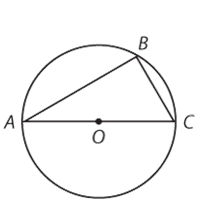In the figure above, the radius of the circle with center O is 1 and BC = 1. What is the area of triangular region ABC?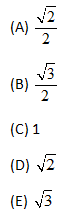What’s your first step? Let’s use this problem as an opportunity to practice the Quant Process.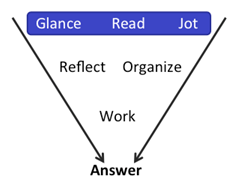At a glance, you can see that the problem provides a diagram. Draw! Make it big enough that you can add labels as you calculate new pieces of information (and, of course, jot down any information given in the problem).

Finally, write down any formulas you’ll need, as well as whatever the problem asks you to find. Your scrap paper might look something like this:

GMAT Challenge Problem Showdown: December 16, 2013We invite you to test your GMAT knowledge for a chance to win! Each week, we will post a new Challenge Problem for you to attempt. If you submit the correct answer, you will be entered into that week’s drawing for a free Manhattan GMAT Prep item. Tell your friends to get out their scrap paper and start solving!

Here is this week’s problem: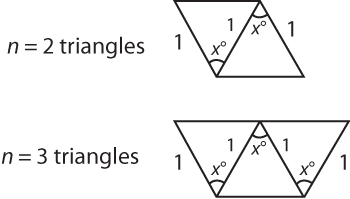A set of n identical triangles with angle x° and two sides of length 1 is assembled to make a parallelogram (if n is even) or a trapezoid (if n is odd), as shown. Is the perimeter of the parallelogram or trapezoid less than 10?

GMAT Challenge Problem Showdown: October 21, 2013We invite you to test your GMAT knowledge for a chance to win! Each week, we will post a new Challenge Problem for you to attempt. If you submit the correct answer, you will be entered into that week’s drawing for a free Manhattan GMAT Prep item. Tell your friends to get out their scrap paper and start solving!

Here is this week’s problem: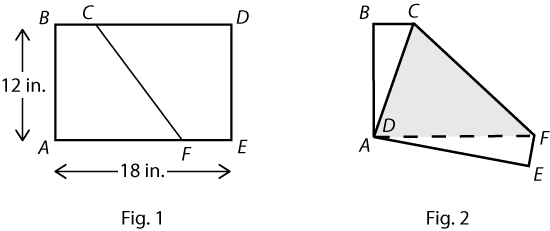A sheet of paper ABDE is a 12-by-18-inch rectangle, as shown in Figure 1. The sheet is then folded along the segment CF so that points A and D coincide after the paper is folded, as shown in Figure 2 (The shaded area represents a portion of the back side of the paper, not visible in Figure 1). What is the area, in square inches, of the shaded triangle shown?

GMAT Challenge Problem Showdown: August 26, 2013We invite you to test your GMAT knowledge for a chance to win! Each week, we will post a new Challenge Problem for you to attempt. If you submit the correct answer, you will be entered into that week’s drawing for a free Manhattan GMAT Prep item. Tell your friends to get out their scrap paper and start solving!
Here is this week’s problem:

If three different integers are selected at random from the integers 1 through 8, what is the probability that the three selected integers can be the side lengths of a triangle?